# Hyperboloid

(diff) ← Older revision | Latest revision (diff) | Newer revision → (diff)

A non-closed central surface of the second order. One distinguishes between two types of hyperboloids: the one-sheet and the two-sheet hyperboloid.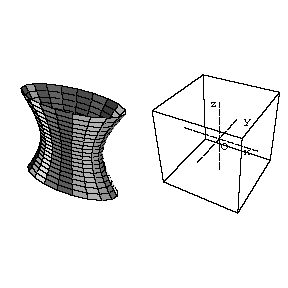Figure: h048360aFigure: h048360b

In a suitable coordinate system (see Fig. a, Fig. b) the equation of a one-sheet hyperboloid is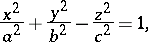while that of a two-sheet hyperboloid isThe numbers,and(and segments of such lengths) are known as the semi-axes of the hyperboloid. Sections of a hyperboloid by planes passing through the-axis are hyperbolas. Sections of a hyperboloid by planes perpendicular to the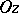-axis are ellipses. The section of a one-sheet hyperboloid by the plane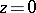is said to be a gorge ellipse. A hyperboloid has three planes of symmetry. The cone defined by the equationis called the asymptotic cone. If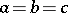, the hyperboloid is said to be regular. A hyperboloid with two equal semi-axes is said to be a hyperboloid of rotation. A one-sheet hyperboloid is a ruled surface; the equations of the rectilinear generators passing through a given pointhave the form